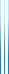# PublicationsManuscripts
We show that the information complexity of the AND function with respect to the distribution $\mu(0,0)=\mu(0,1)=\mu(1,0)=1/3$ is strictly positive for any worst-case error rate (strictly less than $1/2$).
Using this, a simple (but tricky) argument using the chain rule shows that the information complexity of set disjointness is $\Omega(n)$ for any worst-case error rate, which implies that the randomized communication complexity of set disjointness is $\Omega(n)$.